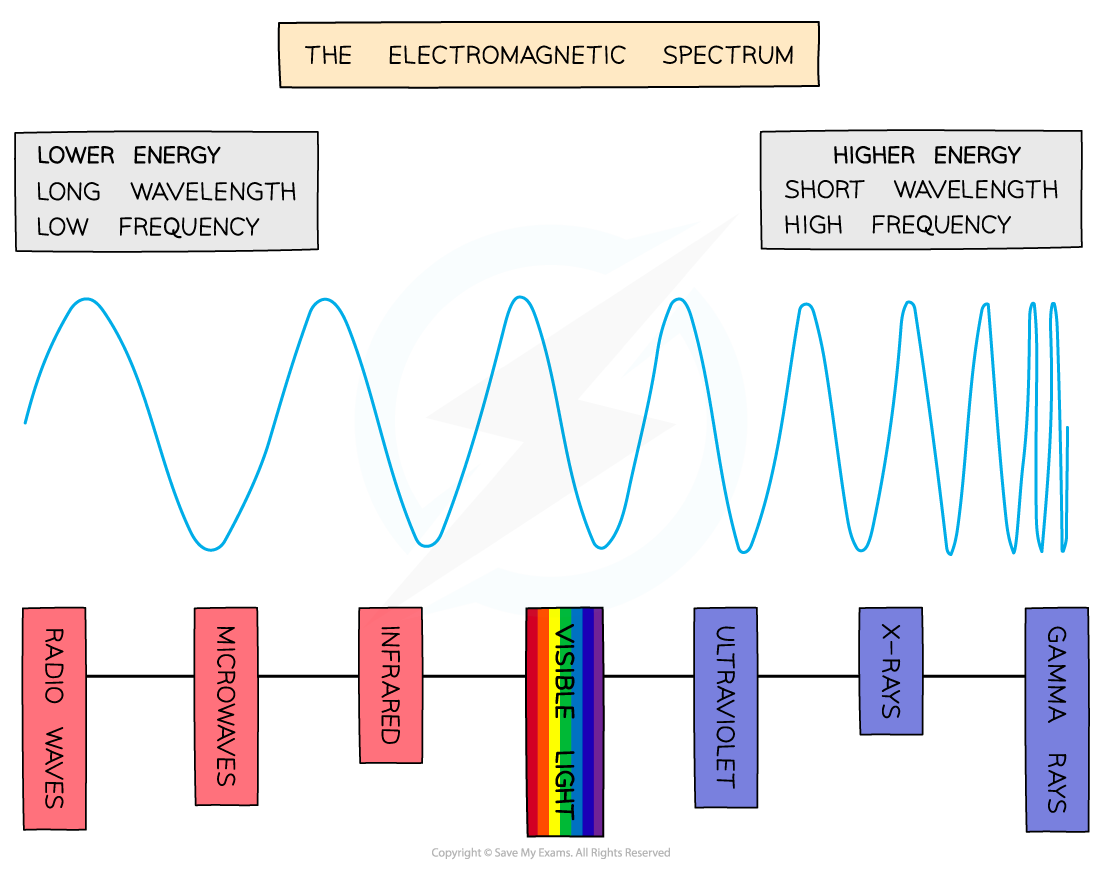# IB DP Chemistry: SL复习笔记2.1.4 The Electromagnetic Spectrum

### The Electromagnetic Spectrum

• The electromagnetic spectrum is a range of frequencies that covers all electromagnetic radiation and their respective wavelengths and energy
• It is divided into bands or regions, and is very important in analytical chemistry
• The spectrum shows the relationship between frequencywavelength and energy
• Frequency is how many waves pass per second, and wavelength is the distance between two consecutive peaks on the wave
• Gamma raysX-rays and UV radiation are all dangerous - you can see from that end of the spectrum that it is high frequency and high energy, which can be very damaging to your health• All light waves travel at the same speed; what distinguishes them is their different frequencies
• The speed of light (symbol ‘c’) is constant and has a value of 3.00 x 108 ms-1
• As you can see from the spectrum, frequency (symbol ‘ν') is inversely proportional to wavelength (symbol ‘λ')
• In other words, the higher the frequency, the shorter the wavelength
• The equation that links them is c = νλ
• Since c is constant you can use the formula to calculate the frequency of radiation given the wavelength, and vice versa

#### Continuous versus line spectrum

• A continuous spectrum in the visible region contains all the colours of the spectrum
• This is what you are seeing in a rainbow, which is formed by the refraction of white light through a prism or water droplets in rainA continuous spectrum shows all frequencies of light

• However, a line spectrum only shows certain frequenciesThe line spectrum of helium which shows only certain frequencies of light

• This tells us that the emitted light from atoms can only be certain fixed frequencies - it is quantised (quanta means 'little packet')
• Electrons can only possess certain amounts of energy - they cannot have any energy value

#### Exam Tip

The formula that relates frequency and wavelength is printed in Section 1 of the IB Chemistry Data Booklet so you don’t need to learn itYou will also find the speed of light and other useful constants in Section 2